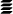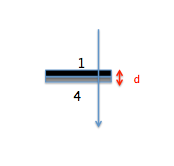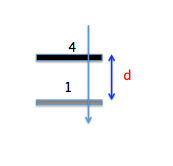You can:  1) Find some help on this page   2) Try it: Step-by-Step Instructions

Muon Speed Using Time of Flight (1)

Using the TOF analysis module, one can measure the average time muons take to travel between two counters. Then, we can calculate average speed (v = d/t). We use the following definitions (same as Flux):

• muon time of arrival at a scintillation counter = hit
• scintillation counter = channel
• 1. We set up the detector and collect data with two counters stacked, one on top of the other.The top counter is connected to channel 1, and the bottom counter is connected to channel 4. Measure from the top of counter 1 to the top of counter 4 to estimate the distance the muon travels (d). Create a histogram similar to the one below, and find the mean time for the muon to travel between counters.Find the mean using the advanced controls. In this case, we find the mean to be -1.74ns and the standard deviation to be 2.59ns. Notice that the mean is slightly negative. What does this mean?

2. The distance between counters, muon's speed, PMT response time, and length of signal cables all affect the time measured between two counters. We must measure the time of flight of the muon that doesn't include those other experimental effects. This requires another data run. Collect data with a larger distance between the counters. How far apart should they be? Recommended: d ~ 2m to begin with. Once again, create a histogram, and find the mean time for muon to travel between the counters.3. Using the results from steps 1 and 2, we can populate the following table:

Run x: Separation (m) y: Mean Traversal Time (mtt) (ns) Std. dev. of mtt (ns) Error on Mean(ns)
1 0.03 -1.74 2.59 0.03
2 2.4 6.31 2.82 0.07

Why do we use the distribution mean?

We now have 2 data points. The x axis represents separation distance, and the y axis represents mean traversal time. We can graph them on paper, use the ScatterPlot tool, or Excel and calculate the slope of the line connecting them. Remember to draw the error bars using mean error. This is 1/(muon speed). Q: What are the units?

Δy / Δx = (6.31 - -1.74)ns/(2.4 - 0.03)m

Therefore, we can say that muon speed = 2.37m/8.05ns = 0.294m/ns = 2.94*10^8m/s.Q: What is the error estimate of muon speed?

4. One can measure muon speed better with more data points representing different separation distances. For example, to generate a third data point, swap the two counters. Now, the separation distance d = -2.4m because the muon travels from counter 4 to counter 1.Run x: Separation (m) y: Mean Traversal Time (mtt) (ns) Std. dev. of mtt (ns) Error on Mean(ns)
1 0.3 -1.74 2.59 0.03
2 2.4 6.31 2.82 0.07
3 -2.4 -9.98 2.94 0.07

We now have 3 data points. Eyeball it, and draw the best fit line. Then, calculate the slope of the line to get a better value for muon speed.

Δy / Δx = 17.0ns/5.0m

Therefore, we find: muon speed = 2.94*10^8m/s

Q: Is this speed close to one that is recognizable?You are now able to measure the average muon speed. You can STOP here, or you can investigate further.

To further improve your measurement, here is another effect to think about. If you use a large separation, the rate of muons going through both counters may be so low that the trigger rate is dominated by the rate of two different muons, neither going through both counters nor traveling the path length we think we are measuring. We do not want to use the times from two different muons since that tells us nothing about the speed of 1 muon hitting both counters.How can you minimize this effect? Go to page 2.

Tutorial Pages: 1 2 3 4 & Analysis